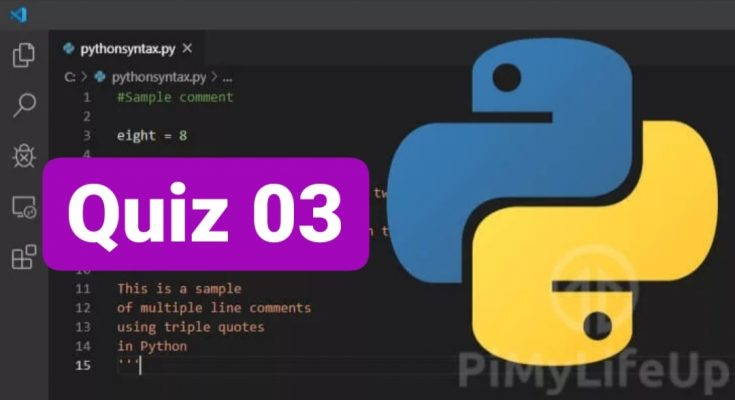# Python Quiz – 03Variable data type is automatically determined by Python. Variables can store data of different types. Python has the following data types built-in by default, in these categories:

1. Text Type: str
2. Numeric Types: int, float, complex
3. Sequence Types: list, tuple, range
4. Mapping Type: dict
5. Set Types: set, frozenset
6. Boolean Type: bool
7. Binary Types: bytes, bytearray, memoryview

# Python Operators

Operators are symbols which are used to perform operations on values and variables. Also, Operators are defined as the constructs which can manipulate the value of operands. The data items are referred as operands or arguments. Operators are either represented by keywords or special characters

1 C : Lists are ordered,indexed and changeable. Similar to Lists, Tuple is an ordered, indexed sequence of items and are immutable i.e items of tuple can not be changed. But, Set is the collection of unordered and unindexed object.

2 C : The boolean data type in python consists of two value i.e. True or False also represented as 0 or 1. Where 0 represents null value.

3 D : Any list, tuple, set, and dictionary are True, except empty ones.

4 C : The data items are referred as operands or arguments.

5 B : +a is unary operator whose value is unchanged.

6 B : +, ** , // are arithmetic operator whereas ^ is bitwise operator

7 B : 3 xor 2 (3^2) is 1 using bitwise XOR operation (3*3 = 9 , typing error)

8 D : All of the above

9 A : The bitwise operators perform bit by bit binary operation on the values of the two operands.

10 A: a = 0100
b = 0101
a&b = 0100 & 0101 = 0100 i.e. 4